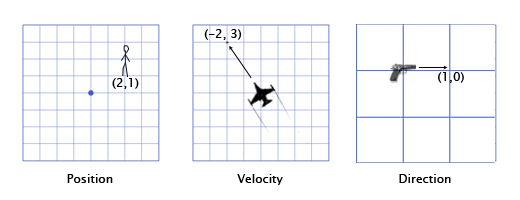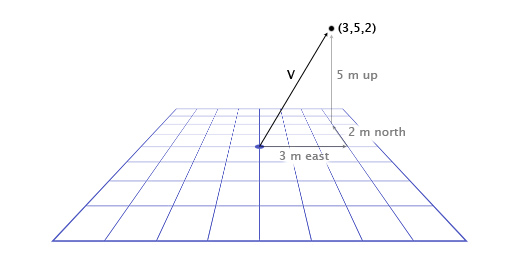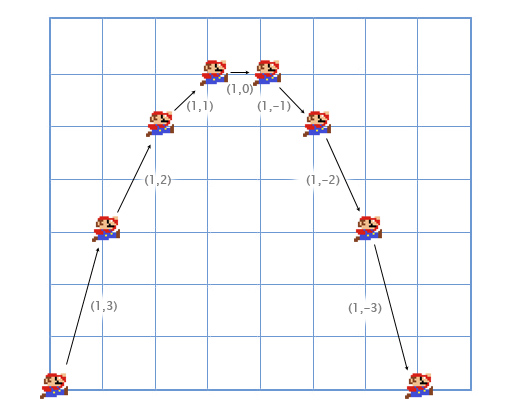# Linear algebra for game developers ~ part 1

July 1st, 2009

When I posted about decals last week, a number of readers commented that they would be interested in posts about linear algebra as it applies to game development. I decided if I'm going to write about that, I might as well start at the beginning! This will be review to many of you who have written games before or taken classes in kinematic physics, so please bear with me for this introductory post -- I will get to more advanced topics later.

### Why do we care about linear algebra?

Linear algebra is the study of vectors. If your game involves the position of an on-screen button, the direction of a camera, or the velocity of a race car, you will have to use vectors. The better you understand linear algebra, the more control you will have over the behavior of these vectors.

### What is a vector?

In games, vectors are used to store positions, directions, and velocities. Here are some 2-Dimensional examples:The position vector indicates that the man is standing two meters east of the origin, and one meter north. The velocity vector shows that in one minute, the plane moves three kilometers up, and two to the left. The direction vector tells us that the pistol is pointing to the right.

As you can see, a vector by itself is just a set of numbers -- it is only given meaning by its context. For example, the vector (1,0) could be the direction for the gun as shown, but it could also be the position of a building one mile to the east of your current position, or the velocity of a snail moving right at a speed of 1 mph.

For this reason, it's important to keep track of your units. Let's say we have a vector V (3,5,2). This doesn't mean much by itself. Three what? Five what? In Overgrowth, positions are always given in meters, and velocities in meters per second. The first number is east, the second is up, and the third is north. Negative numbers represent the opposite directions: west, down, and south. The position represented by (3,5,2) is 3 meters east, 5 meters up, and 2 meters north, as shown here:Now that we've gone over the basics of vectors, we need to know how to use them.

To add vectors together, you just add each component together separately. For example:

(0,1,4) + (3,-2,5) = (0+3, 1-2, 4+5) = (3,-1,9)

Why do we want to add vectors together? One of the most common applications in games for vector addition is physics integration. Any physically-based object will likely have vectors for position, velocity, and acceleration. For every frame (usually 1/60th of a second), we have to integrate these vectors -- that is, add the velocity to the position, and the acceleration to the velocity.

Let's consider the example of Mario jumping. He starts at position (0,0). As he starts the jump, his velocity is (1,3) -- he is moving upwards quickly, but also to the right. His acceleration throughout is (0,-1), because gravity is pulling him downwards. Here is what his jump looks like over the course of seven more frames. The black text specifies his velocity for each frame.We can walk through the first couple frames by hand to see how this works.

For the first frame, we add his velocity (1,3) to his position (0,0) to get his new position (1,3). Then, we add his acceleration (0,-1) to his velocity (1,3) to get his new velocity (1,2).

We do it again for the second frame. We add his velocity (1,2) to his position (1,3) to get (2,5). Then, we add his acceleration (0,-1) to his velocity (1,2) to get (1,1).

Usually in games the player controls a character's acceleration with the keyboard or gamepad, and the game calculates the new velocity and position using physics integration (via vector addition). Fun fact: this is the same kind of integration problem that you solve using integral calculus - we are just using an approximate brute-force approach. I found it much easier to pay attention to calculus classes by thinking about physical applications like this.

### Vector subtraction

Subtraction works in the same way as addition -- subtracting one component at a time. Vector subtraction is useful for getting a vector that points from one position to another. For example, let's say the player is standing at (1,2) with a laser rifle, and an enemy robot is at (4,3). To get the vector that the laser must travel to hit the robot, you can subtract the player's position from the robot's position. This gives us:

(4,3)-(1,2) = (4-1, 3-2) = (3,1).### Scalar-vector multiplication

When we talk about vectors, we refer to individual numbers as scalars. For example, (3,4) is a vector, 5 is a scalar. In games, it is often useful to multiply a vector by a scalar. For example, we can simulate basic air resistance by multiplying the player's velocity by 0.9 every frame. To do this, we just multiply each component of the vector by the scalar. If the player's velocity is (10,20), the new velocity is:

0.9*(10,20) = (0.9*10, 0.9*20) = (9,18).

### Next time

That is all anyone needs to know about vectors to make something like Mario, Pong, or Space Invaders, but there is still a lot left! For part two I would like to get to dot products, cross products, normalization, and reflection, and then part three can be about transformations and vector spaces. Does this make sense so far? Am I going too slow?